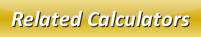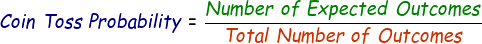# Coin Toss Probability Calculator

Coin toss also known as coin flipping probability is used by people around the world to judge whether its going to be head or tail after flipping the coin. By theory, we can calculate this probability by dividing number of expected outcomes by total number of outcomes.Ā

The formula:For example: If you flip a coin 6 times, what is the probability you get heads twice?

We can find the answer by dividing 2 (expected outcome) by 6 (total outcomes)

= 2/6

= 0.33

So the probability of getting heads twice is 0.33

Similarly, if the aboveĀquestion was to calculate the probability of getting tails then,

6 - 2 = 4

So we can divide 4/6 = 0.66

Therefore, the probability of getting tails is 0.66

Use the below online coin toss probability calculator in similar way. Enter the expected outcomes and total outcomes, press calculate to find out the answer.

 Number of Expected Outcomes: Total Number of Outcomes: Probability:

Latest Calculator Release

Average Acceleration Calculator

Average acceleration is the object's change in speed for a specific given time period. ...

Free Fall Calculator

When an object falls into the ground due to planet's own gravitational force is known a...

Torque Calculator

Torque is nothing but a rotational force. In other words, the amount of force applied t...

Average Force Calculator

Average force can be explained as the amount of force exerted by the body moving at giv...

Angular Displacement Calculator

Angular displacement is the angle at which an object moves on a circular path. It is de...